Next: 4.2 Angles
Up: 4 Lines
Previous: 4 Lines

# 4.1 Distances

The distance between two points in the plane is the length of the line segment joining the two points. If the points have cartesian coordinates (x,y) and (x,y), this distance is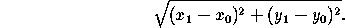If the points have polar coordinates (r,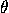) and (r,), this distance is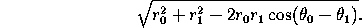If the points have oblique coordinates (x,y) and (x,y), this distance is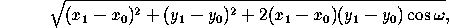where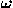is the angle between the axes (Figure 1.5.1 ).

The point k% of the way from P=(x,y) to P=(x,y) is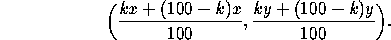(The same formula works also in oblique coordinates.) This point divides the segment PPin the ratio k:(100-k). As a particular case, the midpoint of PPis given by

(½(x+x), ½(y+y)).

The distance from the point (x,y) to the line ax+by+c=0 is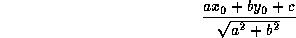Next: 4.2 Angles
Up: 4 Lines
Previous: 4 LinesThe Geometry Center Home Page

Silvio Levy
Wed Oct 4 16:41:25 PDT 1995

This document is excerpted from the 30th Edition of the CRC Standard Mathematical Tables and Formulas (CRC Press). Unauthorized duplication is forbidden.